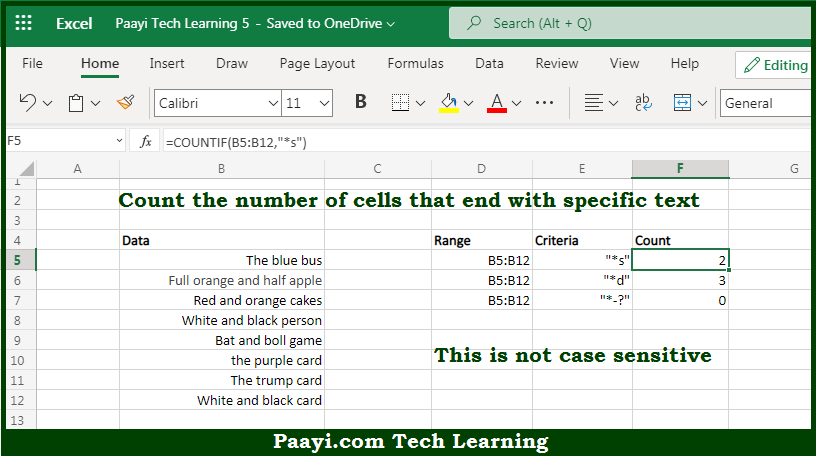# Learn How to Count Cells that End With Specific Text in Microsoft Excel

Written by | 0 Comments | 383 Views

In this article, you will learn how to COUNT various things in Mircosoft Excel using a single or combination of functions and its purpose in Microsoft Excel. You will also get to know how to count cells that end with specific text and see the generic

Count Cells that End With Specific Text in Microsoft Excel

The main purpose of this formula is to count the number of cells that end with the specific text. Here we will learn how to count the range of cells ending with the certain text in Microsoft Excel.  That implies, with the help of a formula based on the  COUNTIF function you can able to count the number of cells in a range that ends with the specific text. So, with the help of this formula, you can able to count the number of cells that have a certain text in the ending.

General Formula to COUNT Cells that End With Specific Text

=COUNTIF(rng,"*txt")

The Explanation for the COUNT Cells that End With Specific TextSo we know that with the help of the given formula above you can able to count the number of cells that end with the specific text. Here we will learn how to count the range of cells ending with the certain text in Microsoft Excel. As we know that, the COUNTIF function is used to count the number of cells in the range that end with "a" by matching the content of each cell against the pattern "*a", which is supplied as the criteria. The "*" symbol r the asterisk is a wildcard in Microsoft Excel which means it can match any number of characters. It should be noted that the count of cells that match this pattern is returned as a number. So, with the help of this formula, you can able to count cells that end with a specific text.